# Euler characteristic facts for kids

Kids Encyclopedia Facts

The Euler characteristic of a shape is a number that describes a topological space, so that anything in the space will have the same number. It is calculated by taking the number of points in the shape, the number of lines in the shape, and the number of faces of the shape. Then, you find the Euler characteristic with this formula: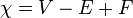$\chi=V-E+F \,\!$

V is the point count, E the line count, and F the amount of faces. For most common shapes, the Euler Characteristic is 2.

Name Image Vertices (Points)
V
Edges (Lines)
E
Faces
F
Euler characteristic:
VE + F
Tetrahedron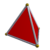4 6 4 2
Hexahedron or cube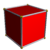8 12 6 2
Octahedron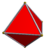6 12 8 2
Dodecahedron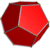20 30 12 2
Icosahedron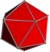12 30 20 2

## Images for kidsEuler characteristic Facts for Kids. Kiddle Encyclopedia.